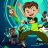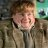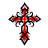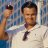# PPO_MMA Indicator for ThinkorSwim

T

#### tomsk

##### Well-known member
VIP
@HighBredCloud @netarchitech if you are interested I have revamped your PPO code so that the moving average of interest are now properly calculated within the code. Please note that I have removed the Ehler's Forward Reverse EMA as that appears to be very EMA specific. Hope this helps you

Code:
``````# PPO Multiple Moving Averages
# Based on earlier PPO FREMAS code from netarchitect
# Revamped by tomsk
# 11.11.2019

declare lower;

input fastPeriod   = 12;
input slowPeriod   = 26;
input signalPeriod = 9;
input price        = close;
input showBreakoutSignals = yes;
input ma_length = 21; #Length(180-200 for floating S/R , 55 for swing entry
input movingAverageType = {"Simple MA", default "Exponential MA", "Wilders Smoothing", "Weighted MA", "Hull MA", "Adaptive MA", "Triangular MA", "Variable MA", "Dema MA", "Tema MA", "EHMA", "THMA"};

def fast;
def slow;

switch (movingAverageType) {
case "Simple MA":
fast = Average(price, fastPeriod);
slow = Average(price, slowPeriod);

case "Exponential MA":
fast = ExpAverage(price, fastPeriod);
slow = ExpAverage(price, slowPeriod);

case "Wilders Smoothing":
fast = WildersAverage(price, fastPeriod);
slow = WildersAverage(price, slowPeriod);

case "Weighted MA":
fast = wma(price, fastPeriod);
slow = wma(price, slowPeriod);

case "Hull MA":
fast = HullMovingAvg(price, fastPeriod);
slow = HullMovingAvg(price, slowPeriod);

case "Triangular MA":
fast = MovAvgTriangular(price, fastPeriod);
slow = MovAvgTriangular(price, slowPeriod);

case "Variable MA":
fast = variableMA(price, fastPeriod);
slow = variableMA(price, slowPeriod);

case "Dema MA":
fast = DEMA(price, fastPeriod);
slow = DEMA(price, slowPeriod);

case "Tema MA":
fast = TEMA(price, fastPeriod);
slow = TEMA(price, slowPeriod);

case EHMA:
fast = ExpAverage(2 * ExpAverage(price, fastPeriod / 2) - ExpAverage(price, fastPeriod), Round(Sqrt(fastPeriod)));
slow = ExpAverage(2 * ExpAverage(price, slowPeriod / 2) - ExpAverage(price, slowPeriod), Round(Sqrt(slowPeriod)));

case THMA:
fast = WMA(WMA(price, (fastPeriod / 2) / 3) * 3 - WMA(price, (fastPeriod / 2) / 2) - WMA(price, (fastPeriod / 2)), (fastPeriod / 2));
slow = WMA(WMA(price, (slowPeriod / 2) / 3) * 3 - WMA(price, (slowPeriod / 2) / 2) - WMA(price, (slowPeriod / 2)), (slowPeriod / 2));
}

def periodOK  = fastPeriod < slowPeriod;
AddLabel(!periodOK, "ERROR: fastPeriod MUST be less than slowPeriod");
def _ppo      = if periodOK then ((fast - slow) / slow) * 100 else 0;
def _signal;

switch (movingAverageType) {
case "Simple MA":
_signal = Average(_ppo, signalPeriod);

case "Exponential MA":
_signal = ExpAverage(_ppo, signalPeriod);

case "Wilders Smoothing":
_signal = WildersAverage(_ppo, signalPeriod);

case "Weighted MA":
_signal = wma(_ppo, signalPeriod);

case "Hull MA":
_signal = HullMovingAvg(_ppo, signalPeriod);

case "Triangular MA":
_signal = MovAvgTriangular(_ppo, signalPeriod);

case "Variable MA":
_signal = variableMA(_ppo, signalPeriod);

case "Dema MA":
_signal = DEMA(_ppo, signalPeriod);

case "Tema MA":
_signal = TEMA(_ppo, signalPeriod);

case EHMA:
_signal = ExpAverage(2 * ExpAverage(_ppo, signalPeriod / 2) - ExpAverage(_ppo, signalPeriod), Round(Sqrt(signalPeriod)));

case THMA:
_signal = WMA(WMA(_ppo, (signalPeriod / 2) / 3) * 3 - WMA(_ppo, (signalPeriod / 2) / 2) - WMA(_ppo, (signalPeriod / 2)), (signalPeriod / 2));
}

plot Ppo      = _ppo;
Ppo.SetDefaultColor(GetColor(1));
Ppo.SetLineWeight(2);

plot PpoEma   = _signal;
PpoEma.SetDefaultColor(GetColor(8));
PpoEma.SetLineWeight(2);

plot zeroLine = 0;
zeroLine.HideBubble();
zeroLine.SetDefaultColor(GetColor(4));

plot PPOdiff  = 2 * (_ppo - _signal);
PPOdiff.SetDefaultColor(GetColor(5));
PPOdiff.SetPaintingStrategy(PaintingStrategy.HISTOGRAM);
PPOdiff.DefineColor("Positive and Up", Color.GREEN);
PPOdiff.DefineColor("Positive and Down", Color.DARK_GREEN);
PPOdiff.DefineColor("Negative and Down", Color.RED);
PPOdiff.DefineColor("Negative and Up", Color.DARK_RED);
PPOdiff.AssignValueColor(if PPOdiff >= 0
then if PPOdiff > PPOdiff
then PPOdiff.Color("Positive and Up")
else PPOdiff.Color("Positive and Down")
else if PPOdiff < PPOdiff
then PPOdiff.Color("Negative and Down")
else PPOdiff.Color("Negative and Up"));
PPOdiff.SetLineWeight(3);

plot UpSignal = if PPOdiff crosses above ZeroLine then ZeroLine else Double.NaN;
plot DownSignal = if PPOdiff crosses below ZeroLine then ZeroLine else Double.NaN;

UpSignal.SetHiding(!showBreakoutSignals);
DownSignal.SetHiding(!showBreakoutSignals);

UpSignal.SetDefaultColor(Color.DOWNTICK);
UpSignal.SetPaintingStrategy(PaintingStrategy.ARROW_UP);
UpSignal.SetLineWeight(2);
DownSignal.SetDefaultColor(Color.UPTICK);
DownSignal.SetPaintingStrategy(PaintingStrategy.ARROW_DOWN);
DownSignal.SetLineWeight(2);
# END CODE``````

Last edited:
•HighBredCloud and netarchitech
T

#### tomsk

##### Well-known member
VIP
@HighBredCloud @netarchitech Here's version 2 of my PPO Multiple Moving Averages study.
This is a revision from an earlier posted version that had two separate switch structures.
I moved some of the definitions around, and collapsed all of this into a single switch statement
Makes future code maintenance easier. Hope this helps

Code:
``````# PPO Multiple Moving Averages
# Based on earlier PPO FREMAS code from netarchitect
# Revamped by tomsk
# 11.13.2019

# V11.11.2019 - tomsk - revamped netarchitect's base PPO code
# V11.13.2019 - tomsk - enhanced code structure, collapsed computation into a single switch

declare lower;

input fastPeriod = 12;
input slowPeriod = 26;
input signalPeriod = 9;
input price = close;
input showBreakoutSignals = yes;
input ma_length = 21; #Length(180-200 for floating S/R , 55 for swing entry
input movingAverageType = {"Simple MA", default "Exponential MA", "Wilders Smoothing", "Weighted MA",
"Hull MA", "Adaptive MA", "Triangular MA", "Variable MA", "Dema MA", "Tema MA", "EHMA", "THMA"};

def periodOK  = fastPeriod < slowPeriod;
AddLabel(!periodOK, "ERROR: fastPeriod MUST be less than slowPeriod");

def fast;
def slow;
def _ppo;
def _signal;

switch (movingAverageType) {
case "Simple MA":
fast = Average(price, fastPeriod);
slow = Average(price, slowPeriod);
_ppo = if periodOK then ((fast - slow) / slow) * 100 else 0;
_signal = Average(_ppo, signalPeriod);

case "Exponential MA":
fast = ExpAverage(price, fastPeriod);
slow = ExpAverage(price, slowPeriod);
_ppo = if periodOK then ((fast - slow) / slow) * 100 else 0;
_signal = ExpAverage(_ppo, signalPeriod);

case "Wilders Smoothing":
fast = WildersAverage(price, fastPeriod);
slow = WildersAverage(price, slowPeriod);
_ppo = if periodOK then ((fast - slow) / slow) * 100 else 0;
_signal = WildersAverage(_ppo, signalPeriod);

case "Weighted MA":
fast = wma(price, fastPeriod);
slow = wma(price, slowPeriod);
_ppo = if periodOK then ((fast - slow) / slow) * 100 else 0;
_signal = wma(_ppo, signalPeriod);

case "Hull MA":
fast = HullMovingAvg(price, fastPeriod);
slow = HullMovingAvg(price, slowPeriod);
_ppo = if periodOK then ((fast - slow) / slow) * 100 else 0;
_signal = HullMovingAvg(_ppo, signalPeriod);

_ppo = if periodOK then ((fast - slow) / slow) * 100 else 0;

case "Triangular MA":
fast = MovAvgTriangular(price, fastPeriod);
slow = MovAvgTriangular(price, slowPeriod);
_ppo = if periodOK then ((fast - slow) / slow) * 100 else 0;
_signal = MovAvgTriangular(_ppo, signalPeriod);

case "Variable MA":
fast = variableMA(price, fastPeriod);
slow = variableMA(price, slowPeriod);
_ppo = if periodOK then ((fast - slow) / slow) * 100 else 0;
_signal = variableMA(_ppo, signalPeriod);

case "Dema MA":
fast = DEMA(price, fastPeriod);
slow = DEMA(price, slowPeriod);
_ppo = if periodOK then ((fast - slow) / slow) * 100 else 0;
_signal = DEMA(_ppo, signalPeriod);

case "Tema MA":
fast = TEMA(price, fastPeriod);
slow = TEMA(price, slowPeriod);
_ppo = if periodOK then ((fast - slow) / slow) * 100 else 0;
_signal = TEMA(_ppo, signalPeriod);

case EHMA:
fast = ExpAverage(2 * ExpAverage(price, fastPeriod / 2) - ExpAverage(price, fastPeriod), Round(Sqrt(fastPeriod)));
slow = ExpAverage(2 * ExpAverage(price, slowPeriod / 2) - ExpAverage(price, slowPeriod), Round(Sqrt(slowPeriod)));
_ppo = if periodOK then ((fast - slow) / slow) * 100 else 0;
_signal = ExpAverage(2 * ExpAverage(_ppo, signalPeriod / 2) - ExpAverage(_ppo, signalPeriod), Round(Sqrt(signalPeriod)));

case THMA:
fast = WMA(WMA(price, (fastPeriod / 2) / 3) * 3 - WMA(price, (fastPeriod / 2) / 2) - WMA(price, (fastPeriod / 2)), (fastPeriod / 2));
slow = WMA(WMA(price, (slowPeriod / 2) / 3) * 3 - WMA(price, (slowPeriod / 2) / 2) - WMA(price, (slowPeriod / 2)), (slowPeriod / 2));
_ppo = if periodOK then ((fast - slow) / slow) * 100 else 0;
_signal = WMA(WMA(_ppo, (signalPeriod / 2) / 3) * 3 - WMA(_ppo, (signalPeriod / 2) / 2) - WMA(_ppo, (signalPeriod / 2)), (signalPeriod / 2));
}

plot Ppo = _ppo;
Ppo.SetDefaultColor(GetColor(1));
Ppo.SetLineWeight(2);

plot PpoEma = _signal;
PpoEma.SetDefaultColor(GetColor(8));
PpoEma.SetLineWeight(2);

plot zeroLine = 0;
zeroLine.HideBubble();
zeroLine.SetDefaultColor(GetColor(4));

plot PPOdiff = 2 * (_ppo - _signal);
PPOdiff.SetDefaultColor(GetColor(5));
PPOdiff.SetPaintingStrategy(PaintingStrategy.HISTOGRAM);
PPOdiff.DefineColor("Positive and Up", Color.GREEN);
PPOdiff.DefineColor("Positive and Down", Color.DARK_GREEN);
PPOdiff.DefineColor("Negative and Down", Color.RED);
PPOdiff.DefineColor("Negative and Up", Color.DARK_RED);
PPOdiff.AssignValueColor(if PPOdiff >= 0
then if PPOdiff > PPOdiff
then PPOdiff.Color("Positive and Up")
else PPOdiff.Color("Positive and Down")
else if PPOdiff < PPOdiff
then PPOdiff.Color("Negative and Down")
else PPOdiff.Color("Negative and Up"));
PPOdiff.SetLineWeight(3);

plot UpSignal = if PPOdiff crosses above ZeroLine then ZeroLine else Double.NaN;
plot DownSignal = if PPOdiff crosses below ZeroLine then ZeroLine else Double.NaN;

UpSignal.SetHiding(!showBreakoutSignals);
DownSignal.SetHiding(!showBreakoutSignals);

UpSignal.SetDefaultColor(Color.DOWNTICK);
UpSignal.SetPaintingStrategy(PaintingStrategy.ARROW_UP);
UpSignal.SetLineWeight(2);
DownSignal.SetDefaultColor(Color.UPTICK);
DownSignal.SetPaintingStrategy(PaintingStrategy.ARROW_DOWN);
DownSignal.SetLineWeight(2);
# END CODE``````

H

#### HighBredCloud

##### Well-known member
VIP
@tomsk Cool...Let me check this out now...ALSO @netarchitech tagged you in another new project...IF you want to help out please feel free...Let us know what you think of the project and what your thoughts are...

H

#### HighBredCloud

##### Well-known member
VIP
@tomsk OK...I just looked at it now...Since I am not a coder...In layman's terms from what I am seeing...It looks and acts the same as version 1 you posted above except you cleaned up the code so its easier to work with for further uses? Am I getting that right?

T

#### tomsk

##### Well-known member
VIP
@HighBredCloud Yes indeed - I streamlined the code, makes it much easier to follow. Functionality same as before, less code statements to deal with so less chance of errors down the road. Regarding the project, let me read through the thread, not real sure what is being requested here. It might help if you could summarize the gist of what you're planning to achieve

•HighBredCloud#### netarchitech

##### Well-known member
VIP
@tomsk Nicely done! Well formed code is a welcome additionThanks for the time and effort spent...much appreciated...

As @HighBredCloud alluded to, take a look, at your leisure, at the latest project and let us know what you think...Thanks!

•HighBredCloud
T

#### tomsk

##### Well-known member
VIP
@netarchitech OK I read through the thread of your latest project. My interpretation is that you'd like the dots to reflect the four states:

Positive and Up
Positive and Down
Negative and Down
Negative and Up

If so you'd need to represent this with 4 different colors and if you look at this visually, some of the colors may not be easily distinguishable.
Hence my input is that you may want it to remain at 2 colors so that at one glance you'll be able to determine the general trend
Not real sure if a 4 color granularity helps with interpretation though. Just my \$0.02

My apologies if I have misunderstood your intent#### netarchitech

##### Well-known member
VIP
@tomsk Thanks for taking a look and swiftly responding...

Yes...preferably 4 states...as we are dealing with 2 histograms (MACD and PPO)...With that said, you make a good point about the color issue...

Would you consider working with us on this project?

My apologies if I have misunderstood your intent
No problem, @tomsk speak freely...you are amongst compatriotsT

#### tomsk

##### Well-known member
VIP
@HighBredCloud @netarchitech Please see my MACD MTF version 2 that incorporates all 4 colors.
Just thought I'd whip a proof of concept up YMMV#### netarchitech

##### Well-known member
VIPPPO (Price Percent Oscillator) for ThinkorSwim Indicators 3The Ringer aka Adaptive RSMK Indicator for ThinkorSwim Indicators 2Z-Score Upper Indicator for ThinkorSwim Indicators 18Aroon Indicator Crossover Arrow? Questions 2